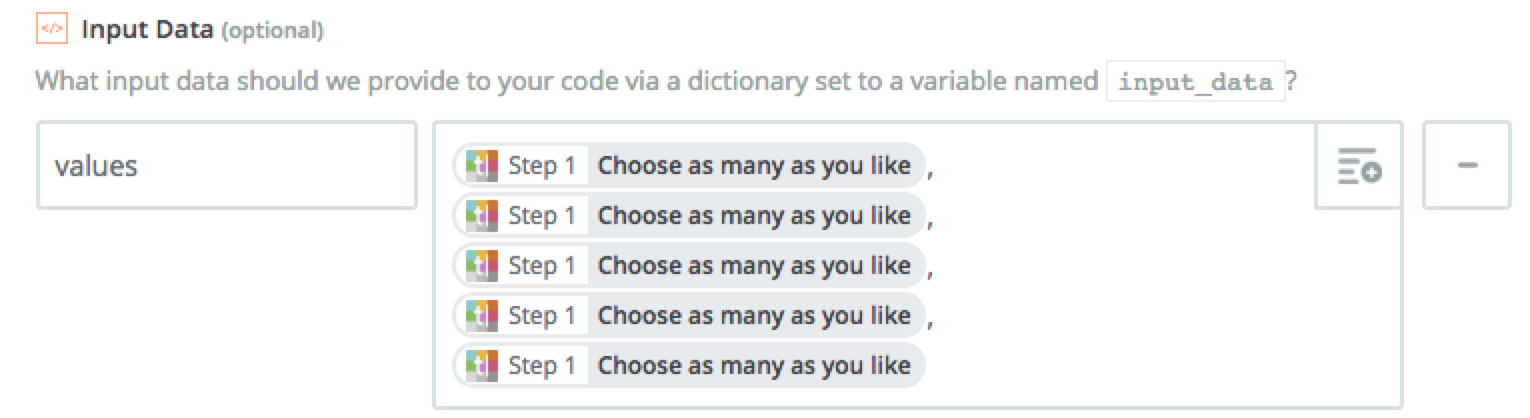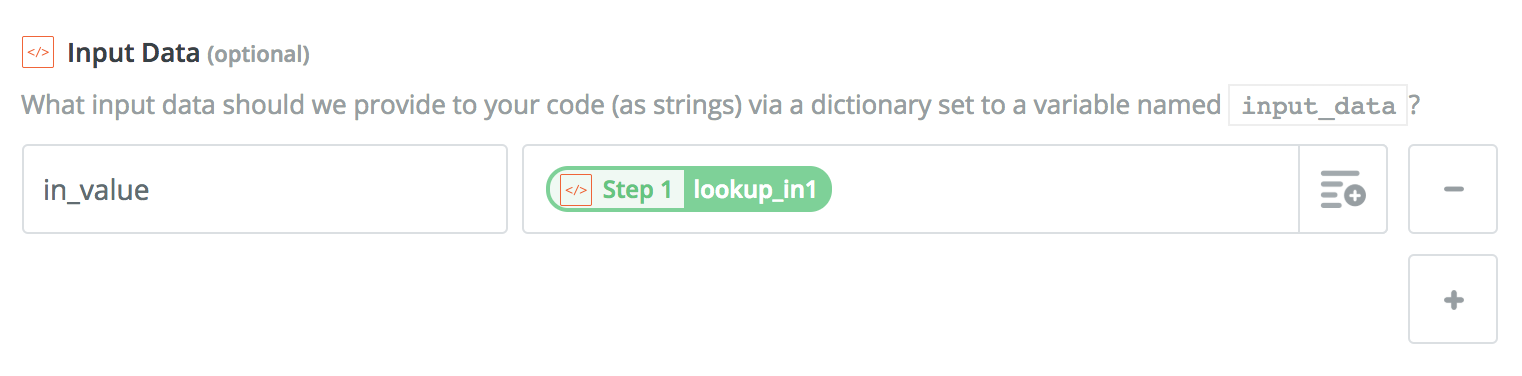# Python code examples in Zaps

This page provides some examples for using Python code steps. Every example depends on specific `input_data`, which is provided under the "Input Data" field when setting up your code step. This example screenshot shows three demonstration inputs you can use in your code like this: `input_data['body']`, `input_data['name']`, and `input_data['subject']`. Be sure you read the code examples and provide the right inputs otherwise nothing will work as expected!Note

Python is an advanced programming language. If you're not familiar with using Python, it's recommended to ask a developer for help.

## Introductory Examples

Each of the four examples below expects a `name` in the "Input Data" field.

A easy example might be something as trivial as:

``return {'id': 1234, 'hello': 'world!', 'name': input_data['name']}``

You can also bind the result to `output`—the code above has exactly the same behavior as the code below:

``output = {'id': 1234, 'hello': 'world!', 'name': input_data['name']}``

An example with an early empty return might be something as trivial as:

``````if input_data['name'] == 'Larry':
return [] # we don't work for Larry!

return {'id': 1234, 'hello': 'world!', 'name': input_data['name']}``````

## Introductory HTTP Example

A more complex example (no "Input Data" needed):

``````response = requests.get('http://example.com/')
response.raise_for_status() # optional but good practice in case the call fails!
return {'id': 1234, 'rawHTML': response.text}``````

## Introductory Logging Example

This example expects a `name` in the "Input Data" field:

``````if input_data.get('name'):
print('got name!', input_data['name'])

return {'id': 1234, 'hello': 'world!', 'name': input_data['name']}``````

Test your action and look at the data to see the `print` result.

## Simple Math - Divide by Two

This example expects a `rawNumber` in the "Input Data" field:

``````return {
'calculatedNumber': int(input_data['rawNumber']) / 2
}``````

## Simple Email Extraction

This example expects a `rawText` in the "Input Data" field:

``````import re
emails = re.findall(r'[\w._-]+@[\w._-]+\.[\w._-]+', input_data['rawText'])
return {
'firstEmail': emails if emails else None
}``````

## Complex Multiple Email Extraction

This example expects a `rawText` in the "Input Data" field:

``````import re
emails = re.findall(r'[\w._-]+@[\w._-]+\.[\w._-]+', input_data['rawText'])
return [
{'email': email} for email in emails
]``````

This will trigger multiple downstream actions—one for each email found! If no emails are found, nothing happens.

## Formatting a Comma Separated List

There may be cases where you need to create a comma separated list but you need to eliminate the blank values from the list. This snippet will help you create the list and remove blanks. This example expects a `values` entry in the "Input Data" field like this:``````import re
values = re.sub('(^,+|,+\$)', '', input_data['values'])
values = re.sub(',+', ',', values)
return [{'values': values}]``````

## Weather JSON API Call

This example expects a `zipCode` in the "Input Data" field:

``````zc = input_data['zipCode']
url = 'http://api.openweathermap.org/data/2.5/weather?zip=' + zc + ',us'
response = requests.get(url)
response.raise_for_status() # optional but good practice in case the call fails!
return response.json()``````

```

## XML Parsing

If you need to parse XML and return it, you can do that with the built in XML libraries:

``````from xml.etree import ElementTree
root = ElementTree.fromstring('''
<parent>
<child><id>1</id></child>
<child><id>2</id></child>
<child><id>3</id></child>
</parent>
''')
return [
{field.tag: field.text for field in child}
for child in root.findall('child')
]``````

If you need to do an API call to parse XML, you can combine this portion with the `request` examples above:

``````from xml.etree import ElementTree
response = requests.get('http://yourapi.com/xml')
root = ElementTree.fromstring(response.text)
return [
{field.tag: field.text for field in child}
for child in root.findall('child')
]``````Note

Please note, the part for iterating the data (`for child in root.findall('child')`) will change depending on whatever the API response looks like, it will not be exactly 'parent' or 'child' like our example above! Experiment with it!

## Store State

It isn't uncommon to want to stash some data away for the next run, our `StoreClient` can do exactly that. For example, this is a counter that counts how many times it is ran:

``````    store = StoreClient('some secret')

count = store.get('some counter') or 0
count += 1
store.set('some counter', count)
return {'the count': count}``````

## Generate Unique ID (UUID)

When you use a Code trigger to connect to an app that uses polling to retrieve new data, your Zap will only trigger if the ID is unique. This is to prevent duplicates.

In the "'hello': 'world!'" example above, the Zap will trigger the first time it receives "'id': 1234". If a later poll retrieves "'id': 1234" again, it will not trigger for that data.

One way to make the ID unique each time the Zap runs is to use the `uuid` library:

``````    import uuid

return {
'unique_id': str(uuid.uuid4())
}``````

## Only Once Per Hour

In conjunction with a Filter step, you can use code like this to limit the number of times your zap can run per hour (which is configurable):

``````every_seconds = 60 * 60

store = StoreClient('some secret')

import time
now = int(time.time())
last_post = store.get('last_post') or now - every_seconds + 1

output = {'run': 'no'}
if last_post < (now - every_seconds):
output = {'run': 'yes'}
store.set('last_post', now)``````

# don't forget to set up a filter after this that checks if run is exactly yesNote

Without a follow up Filter step this won't work!

## Bulk Lookup Table

If you have hundreds of values for lookup, you might not want to use the built-in lookup table tool! This makes it a lot easier, but you'll want to set up the input values like so:``````    default = 'some default'
_lookup_table = '''
​lookup_in1,lookup_out1
​    lookup_in2,lookup_out2
'''.strip().splitlines()
lookup_table = dict(
line.split(',', 1)
for line in _lookup_table.items()
if ',' in line
)
return {
'out_value': lookup_table.get(input_data['in_value'], default)
}``````

Now you can use the "Out Value" in later steps!

0 out of 2 found this helpful
•### Contact Support

Get help from Zapier Support. Contact Support
•### Visit Community

Find answers and inspiration. Visit Community
•### Hire an Expert

Leverage professionals across the globe ready to help. Hire an Expert
•### Zapier University

Video courses designed to help you become a better Zapier user. Zapier University